Back to Adaptive Vision Studio website

You are here: Start » Filter Reference » Geometry 2D Interpolations » LerpVectors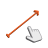# LerpVectors

Linearly interpolates between two vectors.

Name Type Range DescriptioninVector0 Vector2DinVector1 Vector2DinLambda Real -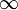-Interpolation between the input vectors where 0.0 value is equal to inVector0 and 1.0 to inVector1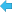outVector Vector2D

### Examples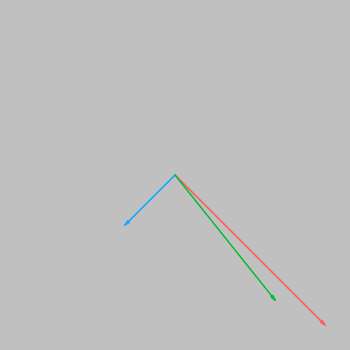LerpVectors performed on red inVector0 = (150, 150) and blue inVector1 = (-50, 50) with inLambda = 0,25. Green is the resulting outVector.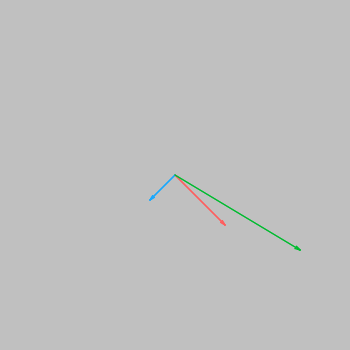LerpVectors performed on red inVector0 = (50, 50) and blue inVector1 = (-25, 25) with inLambda = -1,0. Green is the resulting outVector.

### Remarks

Please note that:
• interpolation begins at inVector0,
• for positive inLambda values interpolation is performed in the direction of inVector1 while for negative - in the direction of a vector which, when visualized with the same base point, ends in the point acquired by mirroring the head of inVector0 by a line perpendicular to the vector (inVector0 - inVector1).
Hence when inLambda = 0,0, outVector is equal to inVector0, while for inLambda = 1,0 it's the same as inVector1 and for inLambda = -1,0 - as the previously described vector. Other inLambda values interpolate between the input vectors and beyond.

### Complexity Level

This filter is available on Basic Complexity Level.

### Filter Group

This filter is member of LerpVectors filter group.